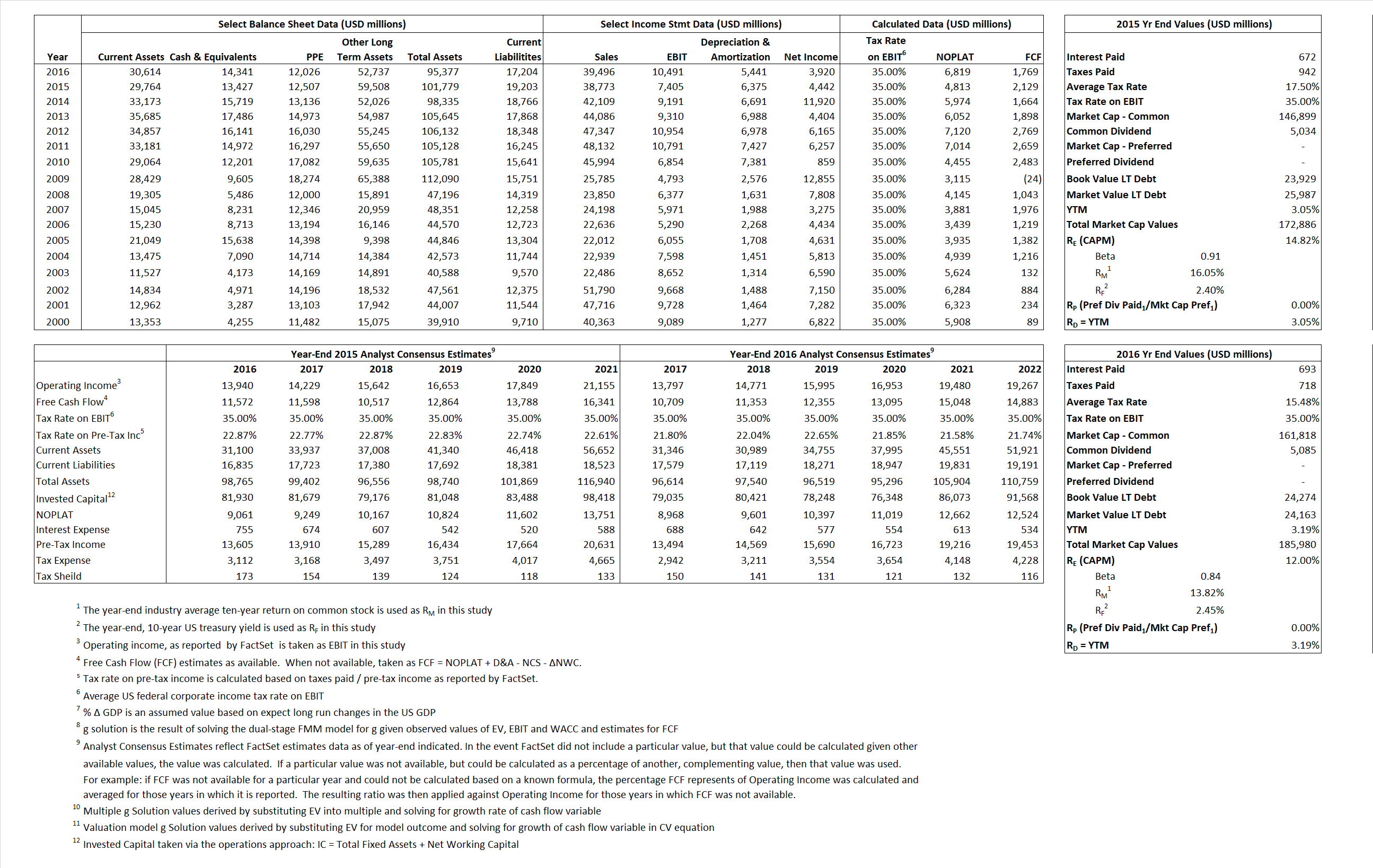# Merck & Co.

## Analyst Listing

The following analysts provide coverage for the subject firm as of May 2016:

 Broker Analyst Analyst Email BMO Capital Markets Alex Arfaei alex.arfaei@bmo.com Berenberg Alistair Campbell alistair.campbell@berenberg.com Morningstar Damien Conover damien.conover@morningstar.com Argus Research David Toung dtoung@argusresearch.com Societe Generale Florent Cespedes florent.cespedes@sgcib.com Deutsche Bank Research Gregg Gilbert tony.butler@guggenheimpartners.com Erste Group Hans Engel hans.engel@erstegroup.com Jefferies Jeffrey Holford jholford@jefferies.com SunTrust Robinson Humphrey John T. Boris john.t.boris@suntrust.com Evercore ISI Mark Schoenebaum mark.schoenebaum@evercoreisi.com Piper Jaffray Richard J. Purkiss richard.j.purkiss@pjc.com Leerink Partners Seamus Fernandez seamus.fernandez@leerink.com Cowen & Company Steve Scala steve.scala@cowen.com Bernstein Research Tim Anderson tim.anderson@bernstein.com Guggenheim Securities Tony Butler tony.butler@guggenheimpartners.com Credit Suisse Vamil Divan vamil.divan@credit-suisse.com

## Primary Input Data## Derived Input Data

### Equational Form

Net Operating Profit Less Adjusted Taxes NOPLAT  4,813  6,819$NOPLAT\, =\, EBIT\, x\, (1 \,-\, Avg \,\,Tax\,\, Rate\,\, on\,\, EBIT)$
Free Cash Flow FCF 2,129 1,769$FCF\,=NOPLAT\,+\,Non-Cash\,Expenses-\Delta NWC\,-\,NCS$
Tax Shield TS 118 107$TS\,=\,Interest\,\,Paid\,\,x\,\, Avg \,\,Tax\,\,Rate\,\, on\,\, Pre-Tax\,\, Income$
Invested Capital IC 82,576 78,173$IC\,=\,Fixed\,\,Operating\,\,Assets\,\,+\,\,Net\,\, Working\,\, Capital$
Return on Invested Capital ROIC 5.83%  8.72%$ROIC\,=\,\frac { NOPLAT }{ IC }$
Net Investment NetInv  9,382  1,038$NetInv\,=\,{ {IC}_{1}}-{{IC}_{0}}+Depreciation$
Investment Rate IR  194.92% 15.22%$IR\,=\,\frac {NetInv}{NOPLAT}$
Weighted Average Cost of Capital
WACCMarket 12.97%  10.79%$WACC\,=\,\frac { E }{ V } { R }_{ E }\,+\,\frac { P }{ V } { R }_{ P }\,+\,\frac { D }{ V } { R }_{ D }\left( 1- Avg\,\, Tax\,\,Rate\,\,on\,\,Pre-Tax\,\,Income \right)$
WACCBook   7.73%   7.21%
Enterprise value
EVMarket  159,459  171,639$EV\,=\,Market\,\,Cap\,\,Equity\,+\,\,Long\,\,Term\,\,Debt\,-\,Cash$
EVBook   156,487   171,751
Long-Run Growth
g = IR x ROIC
11.36%   1.33% Long-run growth rates of the income variable are used in the Continuing Value portion of the valuation models.
g = %$\Delta$ GDP    2.50%   2.50%
Margin from Operations M   19.10%  26.56%$M\,\,=\,\,\frac{EBIT}{SALES}$
Depreciation/Amortization Rate D   46.26%  34.15%$D\,\,=\,\,\frac{D+A}{EBITDA}$

## Valuation Multiple Outcomes

The outcomes presented in this study are the result of original input data, derived data, and synthesized inputs.

### model g solution

12/31/2015 12/31/2016 12/31/2015 12/31/2016 12/31/2015 12/31/2016

EV/SALES$\frac {EV}{Sales} \,= \,\frac{ROIC\, -\, g}{ROIC\,(WACC\,-\,g)}\,(1\,-\,T)\,(M)$

4.11  4.35  20.64%  12.52%  17.39%  11.57%

EV/EBITDA$\frac {EV}{EBITDA} \,= \,\frac{ROIC\, -\, g}{ROIC\,(WACC\,-\,g)}\,(1\,-\,T)\,(1\,-\,D)$

11.57 10.77 20.64% 12.52% 17.39% 11.57%

EV/NOPLAT$\frac {EV}{NOPLAT} \,= \,\frac{ROIC\, -\, g}{ROIC\,(WACC\,-\,g)}$

33.13 25.17 20.64% 12.52% 17.39% 11.57%

EV/FCFOPS$\frac {EV}{FCF_{OPS}} \,= \,\frac{ROIC\, -\, g}{ROIC\,(WACC\,-\,g)}\,(1\,-\,T)$

74.91 97.03 20.64% 12.52% 17.39% 11.57%

EV/EBIT$\frac {EV}{EBIT} \,= \,\frac{ROIC\, -\, g}{ROIC\,(WACC\,-\,g)}\,(1\,-\,T)$

21.53 16.36 20.64% 12.52% 17.39% 11.57%

EV/IC$\frac {EV}{IC} \,= \,\frac{ROIC\, -\, g}{WACC\,-\,g}$

1.93 2.20 20.64% 12.52% 17.39% 11.57%